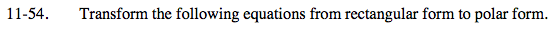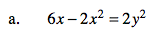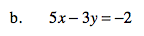Home > PC > Chapter 11 > Lesson 11.1.4 > Problem11-54

11-54.
1. Transform the following equations from rectangular form to polar form. Homework Help ✎

1. 6x − 2x2 = 2y2

2. 5x − 3y = −2$x=r\cos\theta$

$y=r\sin\theta$

$x^2+y^2=r^2$6x = 2x2 + 2y2

6x = 2(x2 + y2)